# Mathematics - Mathematical Analysis Directional Derivatives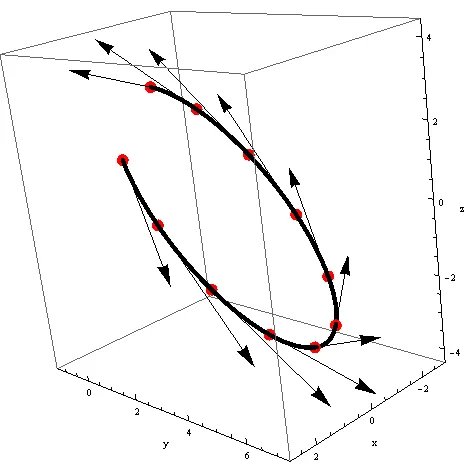Source

## Introduction

Hello it's a me again drifter1!

Today, we will continue with our Mathematical Analysis series of Mathematics by getting into Directional Derivatives that are based on Partial Derivatives that we covered last time.

As I already said last time, Directional Derivatives are used for finding normal vectors to a plane and also for finding tangent planes.

There is not much more to say about, so let's get started!

## Small recap

Last time we talked about Derivatives of vector functions (curves) on top of planes.

If z = f(x, y) and r(t) = x(t)i + y(t)j then the derivative along the curve is given by:

f '(t) = df/dt =  ∂f/∂x * dx/dt + ∂f/∂y * dy/dt

We can see that we use the rule of composition, because those functions are "complex".

The function f(x, y) is a function of x and y, but x(t) and y(t) are functions of t. This means that we first partially differentiate f(x, y) by x or y and multiply this with the derivative of x(t) or y(t) with t.

Of course the derivative along a curve can also be found for 3 or even more dimensions...

## Tangent vectors

Suppose the plane f(x, y, z) = c and the curve r(t) = x(t)i + y(t)j + z(t)k.

The derivative along the curve is:

u = df/dt = ∂f/∂x * dx/dt + ∂f/∂y * dy/dt + ∂f/∂z* dz/dt

The tangent vector of the curve r is: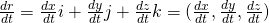quicklatex

The gradient of f (or Del of f) is the vector:

∇f = gradf = (∂f/∂x, ∂f/∂y, ∂f/∂z)

So, the derivative along the curve is:

df/dt = (∂f/∂x, ∂f/∂y, ∂f/∂z) * (dx/dt, dy/dt, dz/dt) = f * u

where ∇f is the gradient of f and u is a tangent vector of curve r(t) = (x(t), y(t), z(t))

If u =(a, b, c) is a unit vector (|u| = 1) and P0 a Point in space then:

f(P0) * u = Duf(P0)

is called the derivative of f along the direction of u = (a, b, c).

For example: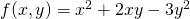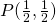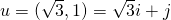Duf(P) = ?

The given vector u is not an unit vector and so we have to convert it.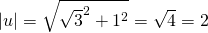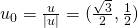∇f = (∂f/∂x, ∂f/∂y) = (2x + 2y, 2x - 6y)

For the point P we have:

∇f(P) = (1 + 1, 1 - 3) = (2, -2)

That way:

Duf(P0) = ∇f(P) * u0 =>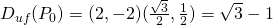Some equations through quicklatex.

## An explanation

Suppose we have the same plane and curve as before, the unit vector u = (u1, u2, u3) and the Point P0(x0, y0, z0).

And ε is a line that passes through P0, is parallel to u and has the parametric equations:

x = x0 + u1t

y = y0 + u2t

z = z0 + u3t

Then dx/dt = u1, dy/dt = u2, dz/dt = u3 and so:

df/dt = ∇f(P0) * (u1, u2, u3) = ∇f(P0) * u = Duf(P0)

Which of course is the derivative of f along the direction of u.

## Some interesting cases

We can write the same equation like that:

Duf(P0) = ∇f(P0) * u = |∇f(P0) ||u|*cosφ

where φ is the angle between the vectors ∇f(P0) and u.

This is clearly a "dot" product between vectors...

Depending on the angle we have the following cases:

1. φ = 0, ∇f(P0) and u are parallel => Duf(P0) = |∇f(P0)| (highest possible increase of f)
2. φ = π, ∇f(P0) = -u => Duf(P0) = -|∇f(P0)| (highest possible decrease of f)
3. φ = π/2, ∇f(P0) and u are across and so Duf(P0) = 0

And so:

-|∇f(P0)| <= Duf(P0) <= |∇f(P0)|

## Normal vectors to a plane

So, now after all that we can finally get into the actual useful thing of this post...

Suppose S: f(x, y , z) = c being a plane/surface and r(t) = x(t)i + y(t)j +z(t)k a curve on top of it that passes through the point P0(x0, y0, z0).

u = dr/dt = (dx/dt, dy/dt, dz/dt) is a tangent vector of curve r at P0.

Let's also suppose N = (a, b, c) being a normal vector to our plane.

Of course we can find a ∇f(P0) so that:

∇f(P0) * u = 0, which means that they are vertically across..

So, N = ∇f(P0)

## Tangent planes

By using this concept, planes/surfaces of the form:

a(x - x0) + b(y- y0) + c(z - z0) = 0

have N = (a, b, c) as a normal vector and also pass through P0.

So, with f(x, y, z) = c being a plane and P0(x0, y0, z0) a point,

the equation of a tangent plane is:

∂f/∂x (x - x0) + ∂f/∂y (y - y0) + ∂f/∂z (z - z0) = 0

For a surface f(x, y) = z we have:

F(x, y, z) = f(x, y) - z = 0

And so;

∂F/∂x = ∂f/∂x

∂F/∂y = ∂f/∂y

∂F/∂z = -1

Which means that:

N = ∇f(P0) = (∂f/∂x, ∂f/∂y, -1)

And finally the equation of such a tangent plane is:

∂f/∂x (x - x0) + ∂f/∂y (y - y0) - (z - z0) = 0

And this is actually it for today and I hope that you enjoyed it!

Next time we will start getting into double/surface integrals!

Bye!

H2
H3
H4
3 columns
2 columns
1 column
1 Comment